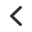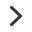August 2019

The assurance of knowing that we are here for you, when and where you need us

The assurance of knowing that we are here for you, when and where you need us

The assurance of knowing that we are here for you, when and where you need us

August 2019

The assurance of knowing that we are here for you, when and where you need us

The assurance of knowing that we are here for you, when and where you need us

The assurance of knowing that we are here for you, when and where you need us